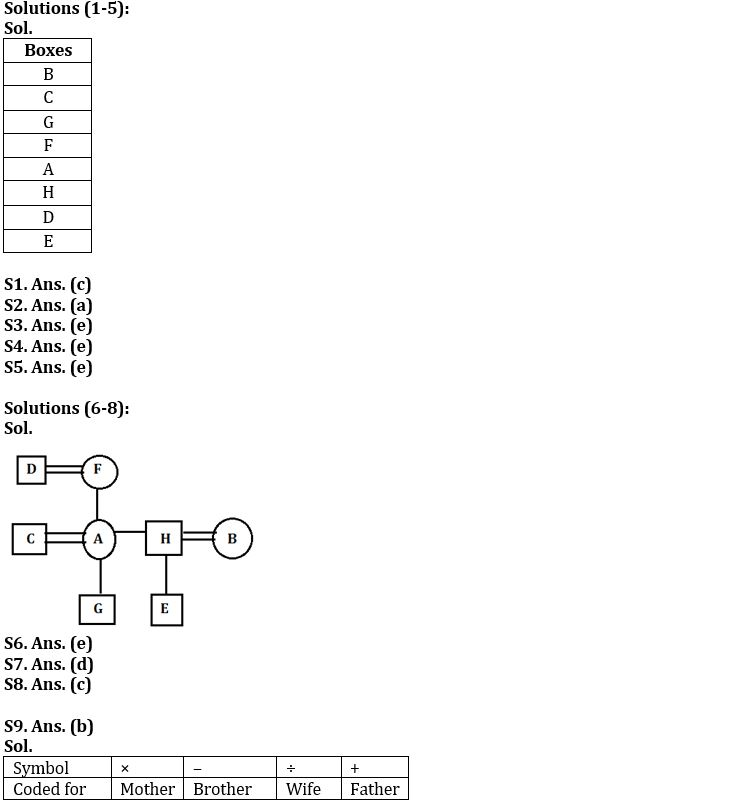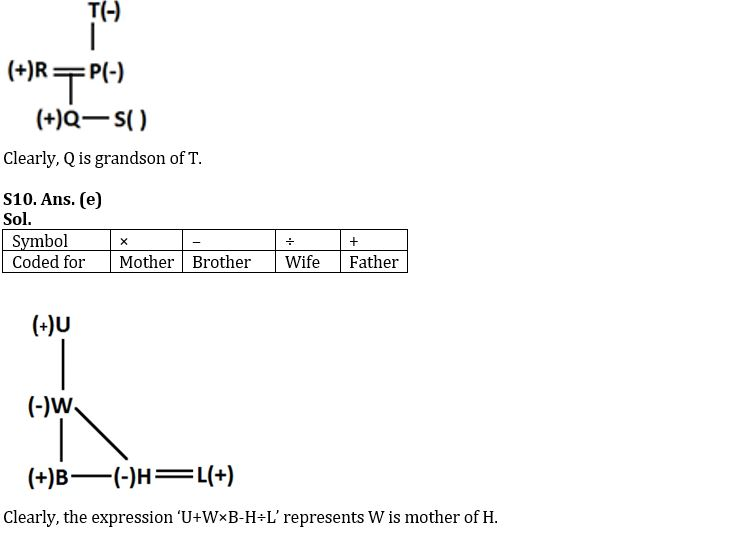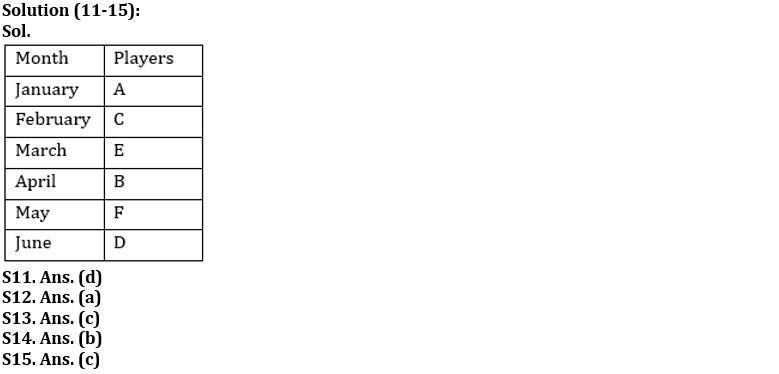Latest Banking jobs   »

# Reasoning Ability Quiz For IBPS Clerk/PO Prelims 2022- 29th August

Directions (1-5): Read the following information carefully and answer the following questions.
Eight boxes A, B, C, D, E, F, G and H are place one above the other in any particular order. Box no. 1 is at the bottom and box no. 8 is at the top. Three boxes are placed between A and B. Box H is placed immediately below A. There are two boxes between H and G. There are as many boxes between C and D as between H and B. Box C is kept above D. Box E is kept immediately below box D. Three boxes are there between E and F.

Q1. How many boxes are there above box D?
(a) Four
(b) Three
(c) Six
(d) Two
(e) None of these

Q2. Which of the following box is kept at the top?
(a) B
(b) A
(c) D
(d) E
(e) None of these

Q3. Choose the odd one out?
(a) B-G
(b) G-A
(c) A-D
(d) C-F
(e) E-D

Q4. Which of the following boxes is kept between F and A?
(a) B
(b) G
(c) C
(d) H
(e) None

Q5. How many boxes are there between C and A?
(a) Less than Two
(b) Four
(c) Five
(d) Six
(e) None of these

Directions (6-8): Study the following information carefully and answer the given questions:
A, D, B, C, E, F, H and G are eight persons of a family. There are three married couple and three generation. A is the only daughter of D, who is the paternal grandfather of E. G is the only child of C. F is mother-in-law of B. The number of males in the family is two more than number of females. E is unmarried. C is the son-in-law of D. H is not married to D.

Q6. How is A related to E?
(a) Mother
(b) Sister
(c) Father
(d) brother
(e) None of these

Q7. How is F related to A?
(a) Son
(b) Father
(c) Daughter
(d) Mother
(e) None of these

Q8. Which of the following is the pair of “husband-wife”?
(a) D, B
(b) C, D
(c) A, C
(d) E, B
(e) F, G

Directions (9-10): Read the following information carefully to answer the following questions-
If ‘P × Q’ means P is mother of Q.
If ‘P – Q’ means P is brother of Q.
If ‘P ÷ Q’ means P is wife of Q.
If ‘P + Q’ means P is father of Q.

Q9. In the expression T×P÷R+Q-S, how is Q related to T?
(a) Daughter
(b) Grandson
(c) Son
(d) Can’t determined
(e) None of these

Q10. In which of the following expression W is mother of H?
(a) U×W+B-H×L
(b) U+W-B÷H+L
(c) U×W÷B-H-L
(d) U÷W+B-H×L
(e) U+W×B-H÷L

Directions (11-15): Read the given information carefully and answer the questions:
Six players are going for tournaments in different months starting from January to June of the same year. A goes in the month which has 31 days. Only two persons are going in between F and C. D goes after F in the month which have even numbers of days. Only two persons are going in between D and E who goes before D. B goes after C who goes before E. Not more than three persons are going in between D and C.

Q11. Who among the following goes immediate before F?
(a) E
(b) C
(c) A
(d) B
(e) None

Q12. Who among the following person going for tournament in February?
(a) C
(b) D
(c) E
(d) F
(e) A

Q13. How many persons are going in between A and F?
(a) None
(b) Four
(c) Three
(d) Two
(e) More than four

Q14. In which month E goes for tournament?
(a) January
(b) March
(c) April
(d) June
(e) February

Q15. Who among the following goes immediate after C?
(a) D
(b) F
(c) E
(d) B
(e) A

Solutions#### Congratulations!Download Hindu Review of October 2021: Free PDF